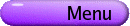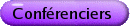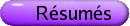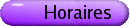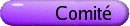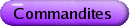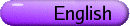Analyse fonctionnelle
Org: Robb Fry (Thompson Rivers) et S. Swaminathan (Dalhousie)
[PDF]

On the number of mutually non-isomorphic infinite-dimensional subspaces of a Banach space
[PDF]

The positive solution to the homogeneous space problem yields that l2 is the only infinite-dimensional Banach space, up to isomorphism, which is isomorphic to all its infinite-dimensional subspaces. For a Banach space X which is not isomorphic to l2, we investigate the problem of finding the number of non-isomorphic infinite-dimensional subspaces of X. As a consequence of our construction we also obtain a structural result about Banach spaces containing an unconditional basic sequence.

JONATHAN BORWEIN, Dalhousie University
Maximality of Sums of Monotone Operators
[PDF]

We say a multifunction T : X ® 2X* is monotone provided that for all x,y Î X, and for all x* Î T(x), y* Î T(y),

 áy-x,y*-x* ñ ³ 0,
and we say that T is maximal monotone if its graph is not properly included in any other monotone graph. The convex subdifferential in Banach space and a skew linear matrix are the canonical examples of maximal monotone multifunctions. Maximal monotone operators play an important role in functional analysis, optimization and partial differential equation theory, with applications in subjects such as mathematical economics and robust control.

In this talk, largely based on , I shall show how-thanks largely to a long-neglected observation of Fitzpatrick-the originally quite complex theory of monotone operators can be almost entirely reduced to convex analysis. I shall also highlight various long-standing open questions to which these new techniques have offered new access, , .

## References


J. M. Borwein, Maximal Monotonicity via Convex Analysis. J. Convex Analysis (special issue in memory of Simon Fitzpatrick), 13(2006), June. [D-drive Preprint 281].


, Maximality of Sums of Two Maximal Monotone Operators in General Banach Space. Proc. Amer. Math. Soc., accepted September 2006. [D-drive Preprint 322].


A. E. Eberhard and J. M. Borwein, Maximality of Monotone Operators in Banach Space. Preprint, September 2006. [D-drive Preprint 325].

MANUEL CEPEDELLO-BOISO, Universidad de Sevilla, Avda. Reina Mercedes s/n, 41012 Sevilla, Spain
Functions with no critical points on separable Banach spaces
[PDF]

The Morse-Sard theorem states that if f : Rn ®Rm is a Cr smooth function, with r > max{n-m,0}, and Cf stands for the set of critical points of f (that is, the points of X at which the differential of f is not surjective), then the set of critical values, f(Cf), is of (Lebesgue) measure zero in Rm. This result is no longer true for functions defined on infinite dimensional Banach spaces. However, under this setting it is possible to develop a strong approximate version of such classical principle.

MAN-DUEN CHOI, University of Toronto
What sort of non-commutative analysis is needed in quantum information theory?
[PDF]

I was totally surprised that all of my earliest research in the 1970s has been used intensively in the recent development of quantum computers. By all means, I need to seek the new meanings of the old values of completely positive linear maps in the setup of non-commutative matrices.

CHANDLER DAVIS, University of Toronto
Subspaces of Hilbert space
[PDF]

The relative position of two 1-dimensional subspaces of Hilbert space is expressed fully by a single number in [0  1]. But suppose we study subspaces P spanned by orthonormal p1, p2, ..., pk and Q spanned by orthonormal q1, q2, ..., qk for higher k. To express the relative position of P and Q fully requires an unordered k-tuple of numbers; this theory has been understood since the 1880s and generalized to infinite-dimensional subspaces. In contrast, it is known that the relative position of three k-dimensional subspaces can not be expressed fully by a manageable invariant.

Nevertheless the manifold of k-subspaces can be studied to advantage. Here is a typical example of a question which can be asked of three subspaces and may have illuminating answers: Given subspaces P, Q, R, is P closer to Q than R is? This talk gives a modern way of dealing with such questions.

MAHIR HASANOV, Istanbul Technical University, Faculty of Science and Letters, Dept. of Mathematics, 34469 Maslak Istanbul, Turkey
On some classes of nonlinear equations in Hilbert spaces arising from the spectral theory
[PDF]

Let L(a) be an operator function of the class C1 ([a,b], S(H) ) such that L(a) << 0, L(b) >> 0 and for all x Î H \{0} the function ( L(a)x, x ) has exactly one zero p(x) in (a,b). Define the following nonlinear operator

Tx = ì
ï
í
ï
î
 L æè p(x) öø x,
 x ¹ 0,
 0,
 x = 0.
We study a connection between solvability problems for the equation Tx=y and completeness problems for eigenvectors of the operator functions L(a). We give some sufficient conditions for completeness of eigenvectors corresponding to eigenvalues from the interval [a, b] which are based on separation properties of the functional p(x).

ALEXANDER LITVAK, University of Alberta
Asymmetry of convex polytopes and vertex index of symmetric convex bodies
[PDF]

In 2002 Gluskin and I showed that a polytope with few vertices is far from being symmetric in a sense of Banach-Mazur distance. More precisely, it was shown that Banach-Mazur distance between such a polytope and any symmetric convex body is large. In this talk we introduce a new, averaging-type parameter to measure the asymmetry. It turns out that, surprisingly, this new parameter is still very large, in fact it satisfies the same lower bound as Banach-Mazur distance. We apply our results to provide a lower estimate on the vertex index of a symmetric convex body, which was recently introduced in by Bezdek and myself. Furthermore, we give the affirmative answer to a conjecture by K. Bezdek on the quantitative illumination problem.

This is joint work with E. D. Gluskin.

GORDON MacDONALD, University of Prince Edward Island
Topologically Transitive Matrix Semigroups
[PDF]

We investigate the properties and structure of topologically transitive multiplicative semigroups of real or complex matrices, and are particularly interested in the question: "What extra conditions must be imposed on such semigroups to guarantee transitivity?"

A set S of matrices is topologically transitive if any non-zero vector can be mapped arbitrarily close to any other vector by a matrix in S, and is transitive if any non-zero vector can be mapped extactly to any other vector by a matrix in S.

This talk is based on joint work with Leo Livshits and Heydar Radjavi.

PETER ROSENTHAL, University of Toronto
Semigroups of matrices; and operators?
[PDF]

I will discuss some results on irreducible semigroups of matrices that Heydar Radjavi and I have recently obtained, and consider which of these results might possibly generalize to semigroups of operators on Hilbert space.

We have established the following: a transitive, closed, homogeneous semigroup of linear transformations on a finite-dimensional space either has zero divisors or is simultaneously similar to a group consisting of scalar multiples of unitary transformations. The proof begins by establishing the result that for each closed homogeneous semi-group with no zero divisors there is a k such that the spectral radius of AB is at most kr(A) r(B) for all A and B in the semigroup. (It is also shown that the spectral radius is not k-submultiplicative on any transitive semigroup of compact operators.)

We have also proven that an irreducible semigroup of complex matrices is, respectively, finite, countable or bounded if there is a non-zero linear functional whose range on the semigroup has the corresponding property. Moreover, an irreducible semigroup is finite, countable or bounded if it has a nonzero ideal with the corresponding property.

I'll discuss as much of the above as time permits.

MOHAMED TAWHID, TRU

KEITH TAYLOR, Dalhousie University, Halifax, NS
Smooth Points in Operator Algebras
[PDF]

We investigate smooth points (points where the norm is differentiable) in C*-algebras and von Neuman algebras. The goal is to obtain characterizations that connect to the operator algebra structure.

This talk is based on joint work with Wend Werner.

VICTOR ZAKHAROV, Institute of Continuum Mechanics of RAS, 1, Acad. Korolyov Street, 614013, Perm, Russia
Construction of compactly supported wavelets adapted to differential operators
[PDF]

We present constructive methods to obtain compactly supported biorthogonal wavelets adapted to differential (pseudodifferential) operators. Such wavelets provide a diagonal form of the corresponding operators and can be useful for numerical applications. Note that the construction of the wavelets boils down mainly to obtain the corresponding masks. Note also that operator-adapted wavelets are closely connected with wavelet bases for some functional spaces (in particular, Sobolev's spaces). We introduce an original approach to obtain wavelets on an interval adapted to monomial differential operators. A generalization to sum of differential operators is discussed. We present a method to adapt wavelets to differential operators with polynomial coefficients. In the multidimensional case we introduce a construction of wavelets adapted to hyperbolic differential operators. A preliminary idea on how to adapt wavelets to the Laplace operator is also considered. Note that, in general, operator-adapted wavelets are not shift and scale invariant (second generation wavelets) and this raises the problem of the well-posedness of the corresponding Multiresolution Analyses. We also discuss a conceptual possibility of adapting frames to operators.